Courses

# NEET Previous Year Questions (2014-20): Equilibrium Notes | EduRev

## Class 11 : NEET Previous Year Questions (2014-20): Equilibrium Notes | EduRev

The document NEET Previous Year Questions (2014-20): Equilibrium Notes | EduRev is a part of the Class 11 Course Chemistry Class 11.
All you need of Class 11 at this link: Class 11

Q.1. Find out the solubility of Ni(OH)2 in 0.1 M NaOH. Given that the ionic product of Ni(OH)2 is 2×10–15.     (2020)
(a) 1 × 10-13 M
(b) 1 × 108 M

(c) 2 × 10-13 M
(d) 2 × 10-8 M

Ans: c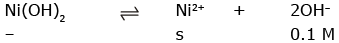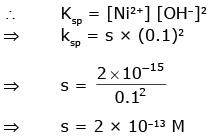Q.2. HCl was passed through a solution of CaCl2, MgCl2 and NaCl. Which of the following compound(s) crystallise(s)?     (2020)
(a) only MgCl2
(b) NaCl, MgCl2 and CaCl2
(c) Both MgCl2 and CaCl2
(d) Only NaCl
Ans:
a
MgCl2 has least solubility among CaCl2, MgCl2 & NaCl thats why it crystallise first on passing HCl.

Q.3. pH of a saturated solution of Ca(OH)2 is 9. The solubility product (Ksp) of Ca(OH)2 is:    (2019)
(a) 0.5 × 10-15
(b) 0.25 × 10-10
(c) 0.125 × 10-15
(d) 0.5 × 10-10
Ans:
a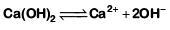pH = 9 Hence pOH = 14 - 9 = 5
[OH-] = 10-5 M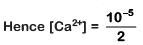Thus Ksp = [Ca2+][OH-]2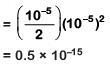Q.4. Conjugate base for Brönsted acids H2O and HF are :    (2019)
(a) OH- and H2F+, respectively
(b) H3O+ and F-, respectively
(c) OHand F-, respectively
(d) H3O+ and H2F+, respectively
Ans:
c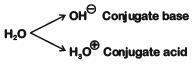HF on loss of Hion becomes Fis the conjugate base of HF
Example :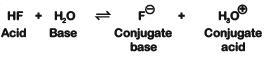Q.5. Which will make basic buffer?    (2019)
(a) 50 mL of 0.1 M NaOH + 25 mL of 0.1 M CH3COOH
(b) 100 mL of 0.1 M CH3COOH + 100 mL of 0.1 M NaOH
(c) 100 mL of 0.1 M HCl + 200 mL of 0.1 M NH4OH
(d) 100 mL of 0.1 M HCl + 100 mL of 0.1 M NaOH
Ans:
c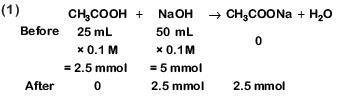This is basic solution due to NaOH.
This is not basic buffer.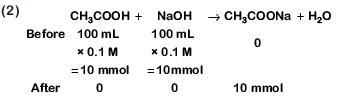Hydrolysis of salt takes place.
This is not basic buffer.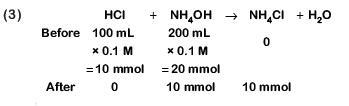This is basic buffer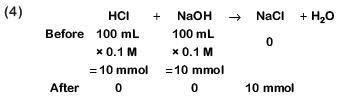⇒ Neutral solution

Q.6. Following solutions were prepared by mixing different volumes of NaOH and HCl of different concentrations :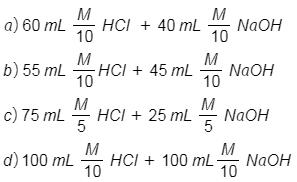pH of which one of them will be equal to 1 ?    (2018)
(1) b
(2) a
(3) d
(4) c

Ans: d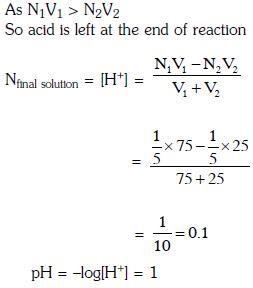Q.7. The solubility of BaSO4 in water 2.42×10gL-1 at 298 K. The value of solubility product (Ksp) will be(Given molar mass of BaSO4 = 233 g mol-1)    (2018)
(a) 1.08 × 10-10 mol2 L-2
(b) 1.08 × 10-12 mol2 L-2
(c) 1.08 × 10-14 mol2 L-2
(d) 1.08 × 10-8 mol2 L-2
Ans:
a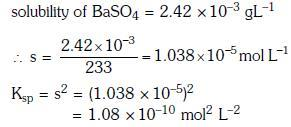Q.8. Which one of the following conditions will favour maximum formation of the product in the reaction
A2(g) + B2(g) ⇌ X2(g) ΔrH = -X kJ ?    (2018)
(a) Low temperature and high pressure
(b) Low temperature and low pressure
(c) High temperature and high pressure
(d) High temperature and low pressure
Ans:
a
For reaction ΔH = - ve and Δng = - ve
∴ High P, Low T, favour product formation.

Q.9. The equilibrium constant of the following are :    (2017)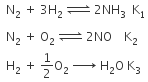The equilibrium constant (K) of the reaction: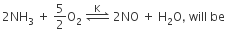(a)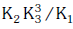(b)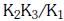(c)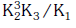(d)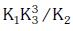Ans:
a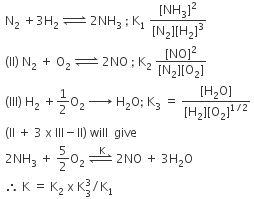Q.10. Concentration of the Ag+ ions in a saturated solution of Ag2C2O4 is 2.2 × 10-4 mol L-1 Solubility product of Ag2C2O4 is :-    (2017)
(a) 2.66 × 10-12
(b) 4.5 × 10-11
(c) 5.3 × 10-12
(d) 2.42 × 10-8
Ans:
c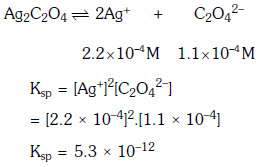Q.11. Which one of the following statements is not correct?    (2017)
(a) The value of equilibrium constant is changed in the presence of a catalyst in the reaction at equilibrium
(b) Enzymes catalyse mainly bio-chemical reactions
(c) Coenzymes increase the catalytic activity of enzyme
(d) Catalyst does not initiate any reaction
Ans:
a
Equilibrium constant is not affected by presence of catalyst.
Hence, statement (1) is incorrect.

Q.12. A 20 litre container at 400 K contains CO2(g) at pressure 0.4 atm and an excess of SrO (neglect the volume of solid SrO). The volume of the container is now decreased by moving the movable piston fitted in the container. The maximum volume of the container, when pressure of CO2 attains its maximum value, will be :-    (2017)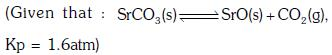(a) 10 litre
(b) 4 litre
(c) 2 litre
(d) 5 litre
Ans
: d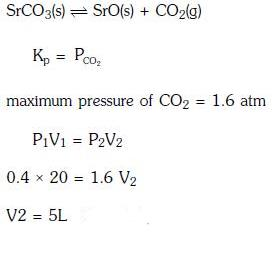Q.13. The addition of a catalyst during a chemical reaction alters which of the following quantities ?    (2016)
(a) Activation energy
(b) Entropy
(c) Internal energy
(d) Enthalpy
Ans:
a
Catalyst can affect only activation energy of the chemical reaction and cannot alter any thermodynamic parameters :
(ie. ΔH , ΔG , ΔS )

Q.14. MY and NY3, two nearly insoluble salts, have the same Ksp values of 6.2 x 10-13 at room temperature, which statements would be true in regard to MY and NY3?    (2016)
(a) The addition of the salt of KY to solution of MY and NY3 will have no effect on their solubilities.
(b) The molar solubilities of MY and NY3 in water are identical.
(c) The molar solubility of MY in water is less than that of NY3.
(d) The salts MY and NY3 are more soluble in 0.5 M KY than in pure water.
Ans:
c
For MY,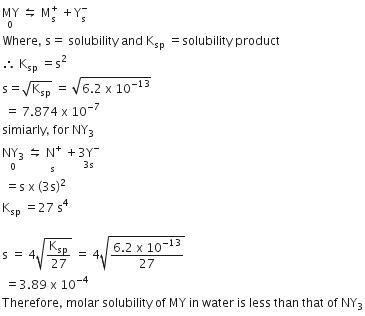Q.15. The Ksp of Ag2CrO4, AgCl, AgBr and AgI are respectively, 1.1 x 10-12, 1.8 x 10-10, 5.0 x 10-13, 8.3 x 10-17. Which one of the following salts will precipitate last if AgNO3 solution is added to the solution containing equal moles of NaCl, NaBr, NaI and Na2CrO4 ?    (2015)
(a) Ag2CrO4
(b) AgI
(c) AgCl
(d) AgBr
Ans:
a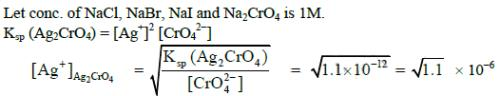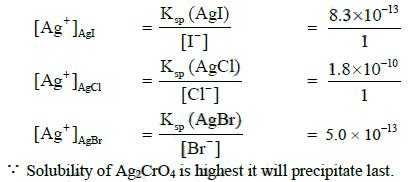Q.16. If the value of an equilibrium constant for a particular reaction is 1.6 x 1012, then at equilibrium the system will contain :    (2015)
(a) similar amounts of reactants and products
(b) all reactants
(c) mostly reactants

(d) mostly products
Ans:
d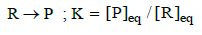Q.17. For the reversible reaction :
N2(g) + 3H2(g) ⇔ 2NH3 (g) + heat
The equilibrium shifts in forward direction :    (2014)
(a) by decreasing the concentrations of N2(g) and H2(g)
(b) by increasing pressure and decreasing temperature
(c) by increasing the concentration of NH3(g)
(d) by decreasing the pressure

Ans: b
As the forward reaction is exothermic and leads to lowering of pressure (produces lesser number of gaseous moles). Hence, According to Le Chatelier's principle, at high pressure and low temperature, the given reversible reaction will shift in forward direction to form more product.

Q.18. Using the Gibbs energy change, ΔG° = +63.3 kJ, for the following reaction,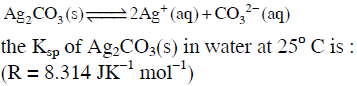(2014)
(a) 2.9 × 10-3
(b) 7.9 × 10-2
(c) 3.2 × 10-26
(d) 8.0 × 10-12
Ans:
d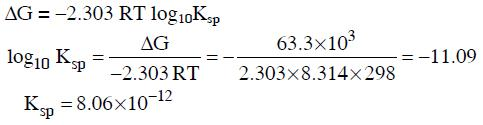Q.19. Which of the following salts will give highest pH in water ?    (2014)
(a) Na2CO3
(b) CuSO4
(c) KCl
(d) NaCl
Ans:
a
The highest pH refers to the basic solution containing OH- ions. Therefore, the basic salt releasing OH- ions on hydrolysis will give highest pH in water.
Only the salt of a strong base and weak acid would release OHion on hydrolysis. Among the given salts, Na2CO3 corresponds to the basic salt as it is formed by the neutralisation of NaOH [strong base] and H2CO3 [weak acid]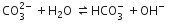Q.20. For a given exothermic reaction, Kp and K'p are the equilibrium constants at temperatures T1 and T2 , respectively. Assuming that heat of reaction is constant in temperature range between T1 and T2 , it is readily observed that :    (2014)
(a)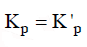(b)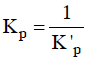(c)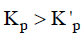(d)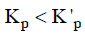Ans:
c
For exothermic reactions, on increasing the temperature the value of equilibrium constant decreases.

Offer running on EduRev: Apply code STAYHOME200 to get INR 200 off on our premium plan EduRev Infinity!

## Chemistry Class 11

129 videos|213 docs|188 tests

,

,

,

,

,

,

,

,

,

,

,

,

,

,

,

,

,

,

,

,

,

;